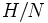# Subquotient

## Contents

VIEW RELATED: Analogues of this | Variations of this | Opposites of this |
View a complete list of semi-basic definitions on this wiki

## Definition

### Symbol-free definition

A subquotient of a group is a quotient of a subgroup of the group by a normal subgroup of that subgroup.

### Definition with symbols

Let$G$ be a group. A subquotient of$G$ is a group of the form$H/N$ where$H$ is a subgroup of$G$ and$N$ is a normal subgroup of$H$.

## Alternative names

Subquotients are also called: